### Home > CALC > Chapter 7 > Lesson 7.1.5 > Problem7-52

7-52.
1. As Khalid inflates a spherical balloon, Kareem wonders about its different rates. He knows that the rate at which Khalid blows is equal to the rate at which the volume changes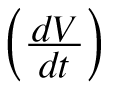. As the balloon inflates, other aspects are changing as well, such as the radius and the surface area. Homework Help ✎

1. If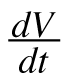= 10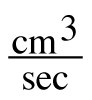, find the rate of change of the radius,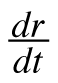, when r = 3 cm.

2. If= 12, find the rate of change of the surface area,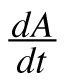, when r = 5 cm.

3. Describe what happens to the balloon whenis negative.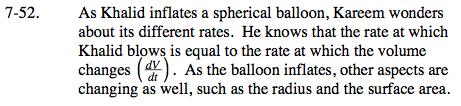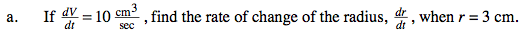Using just geometry (no Calculus), find the equation for a generic spherical balloon.

$V=\frac{4}{3}\pi r^{3}$

Substitute the given information and solve.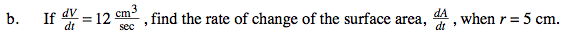$\text{Find }\frac{dr}{dt}, \text{ you will need that information later.}$

Using just geometry, write the equation for the Surface Area of a generic spherical balloon: A = 4πr²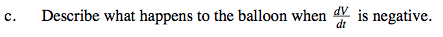What does a negative rate represent?